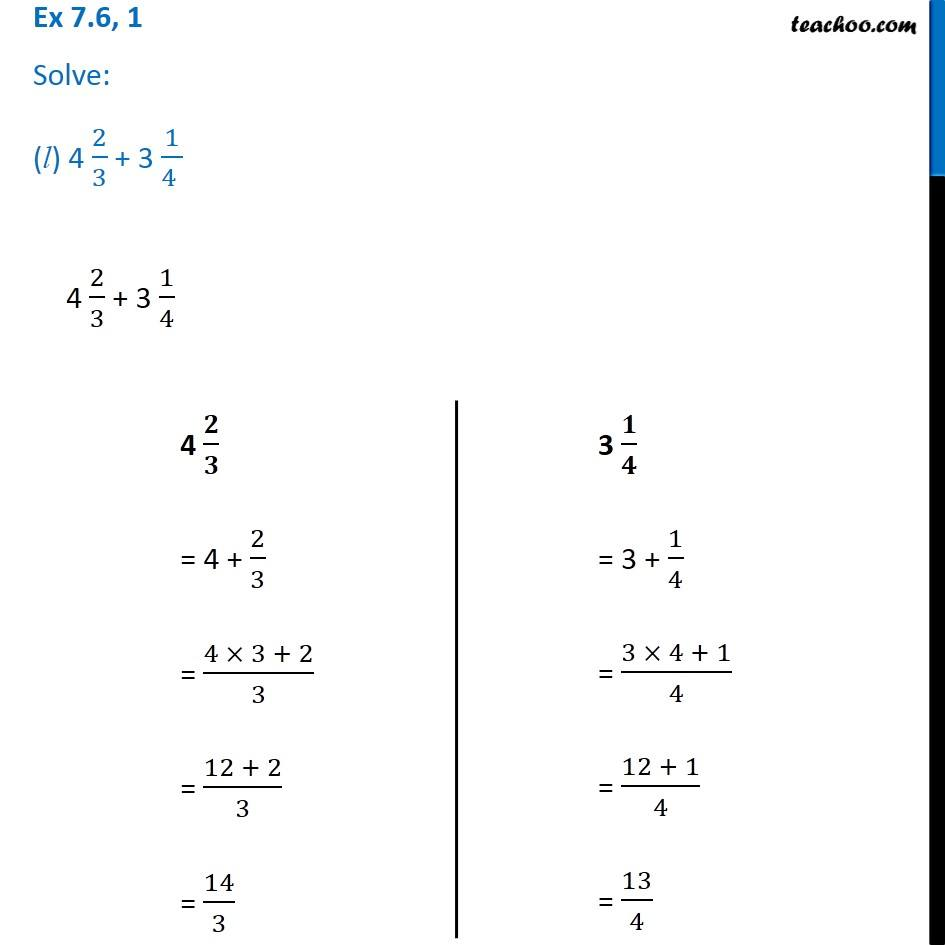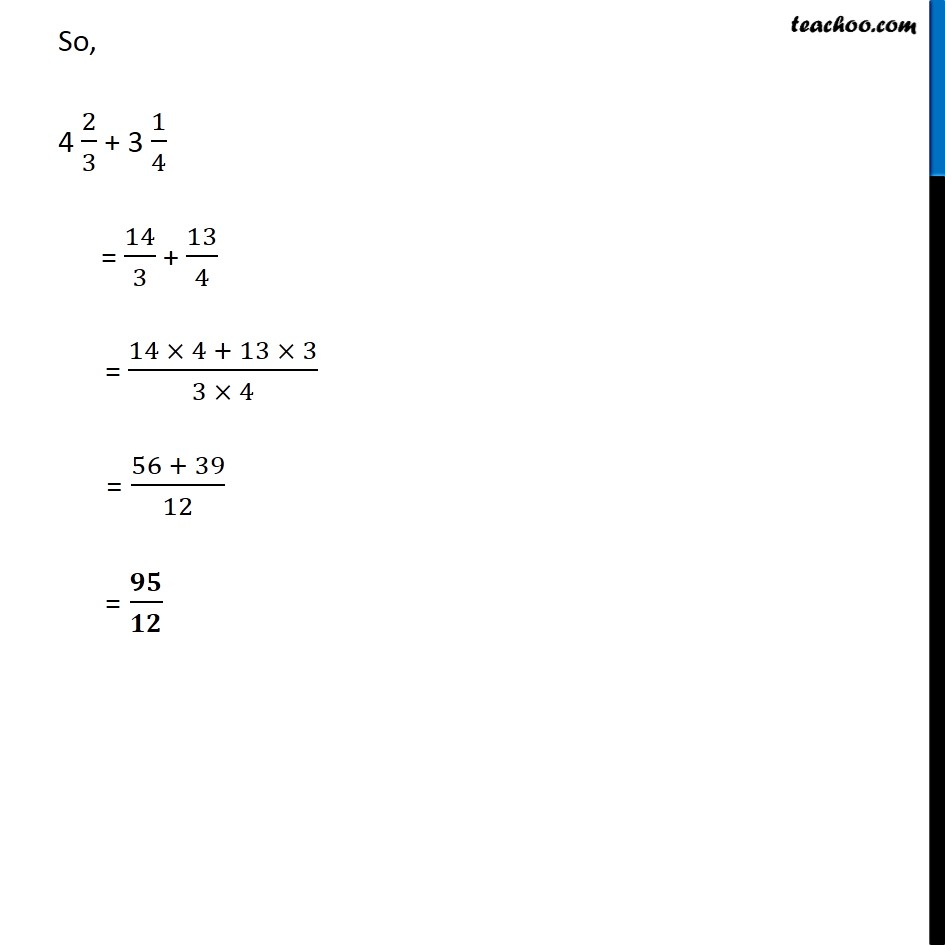1. Chapter 7 Class 6 Fractions
2. Serial order wise
3. Ex 7.6

Transcript

Ex 7.6, 1 Solve: (l) 4 2/3 + 3 1/(4 ) Ex 7.6, 1 Solve: (l) 4 2/3 + 3 1/(4 ) Ex 7.6, 1 Solve: (l) 4 2/3 + 3 1/(4 ) 4 2/3 + 3 1/4 4 / = 4 + 2/3 = (4 3 + 2)/3 = (12 + 2)/3 = 14/3 3 / = 3 + 1/4 = (3 4 + 1)/4 = (12 + 1)/4 = 13/4 So, 4 2/3 + 3 1/4 = 14/3 + 13/4 = (14 4 + 13 3)/(3 4) = (56 + 39)/12 = /